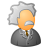# QlikView App Dev

Discussion Board for collaboration related to QlikView App Development.

cancel
Showing results for
Did you mean:Creator

## Set analysis to variables

Hi all,

I need to use a variable and  filter it with set analysis (in a pivot table) and I cant come to how to do it.

The variable is the calculation of a metric(Performance), all the fields of a particular dimension (Product Line) have one vale of the metric associeted. What i need is to calculate the average performance for 3 specific product lines (A , B , C), the 3 product lines together must be represented with 1 single name 'Total GSS' which its performance= avg(performance A , B, ,C ).

The variable is the following:

vCSAT=sum(TCE_RSLT_METRIC_CSAT_FLAG*if(vWithWeights=1,TCE_RSLT_WEIGHTS,1))/sum(TCE_RSLT_METRIC_CSAT_DSAT_TOTAL_FLAG*if(vWithWeights=1,TCE_RSLT_WEIGHTS,1));

As a dimension I am using:

'GSS'

expression:

// This is just a tryal, that is why i am only tring to filter by 1 Product Line. Nevertheless the objective is the descrived above.

avg({\$<Product Line='A'>} \$(vCSAT))

Thanks a lot for you time and help.

If something is not clear just ask it and i will answer asap.

Regards,

Lluís ^^

25 RepliesCreator
Author

No, it is just a random number.Partner

It's hard to provide an answer on a random number.

Have a look at this example and let me know if I am anywhere near where your answer should be.

Really without a target I'm working in the dark.

Good luck

OscarMaster II

sum({< TCE_RSLT_METRIC_CSAT_FLAG >}TCE_RSLT_METRIC_CSAT_FLAG) +

sum({< TCE_RSLT_METRIC_CSAT_DSAT_TOTAL_FLAG>}TCE_RSLT_METRIC_CSAT_DSAT_TOTAL_FLAG)

this would give you combined result if it makes sense but for me it is still confusing.

Find the attachment.Master II

finally!Creator
Author

Yes!! This is working!! I think that it wasn't working for me before becouse i just put 'GSS' (as a text in the dimension) and you defined it in the script.

Thank you very much for all you time and dedication, you helped me a lot !

Regards!

Lluís

Ps. Sorry for being messy i amb a begginer to qlik and to comunities like this ^^.Creator
Author

Just for the record, and if someone is still interested i can explain further and better, i have come to this solution (using the solution you provided me here):

-Defining a variable vCSAT_GSS:

vCSAT_GSS = sum({\$<[Product Line]={'A','B','C'}>}TOTAL TCE_RSLT_METRIC_CSAT_FLAG)/sum({\$<[Product Line]={'A','B','C'}>}TOTAL TCE_RSLT_METRIC_CSAT_DSAT_TOTAL_FLAG)

-Creating a table with the dimension 'GSS' (synthetic, is just a string).

-In the EPRESSION tab just calling the variable \$(vCSAT_GSS)

Done! ^^

Another solution is:

-Creating a table with the dimension 'GSS' (synthetic, is just a string).

-In the EPRESSION tab: =avg({\$<Product line={'A','B','C'}>}TOTAL<GEO> AGGR(\$(vCSAT), [Product Line]))

With this there is no need to create the dimension in the load file.

Pd. The result is a little bit different but the main thing is solved anyway. As I said if you are still interested i can explain further, but now, i don't have much time#Qlikisdarkandfulloftricks